# How to use the intersection operator in Microsoft ExcelThe intersection operator is one of the main operators in Microsoft Excel, but only a small number of users are familiar with it. In this article, I will correct this situation and explain in detail what this operator is and in what situations it can be used.

Pay attention to the following screenshot. On it you see a table with columns and rows. A column with a row is highlighted, and there is a cell in which they intersect. The intersection operator and is needed to find this cell. By default, it will output the exact value that is in that location.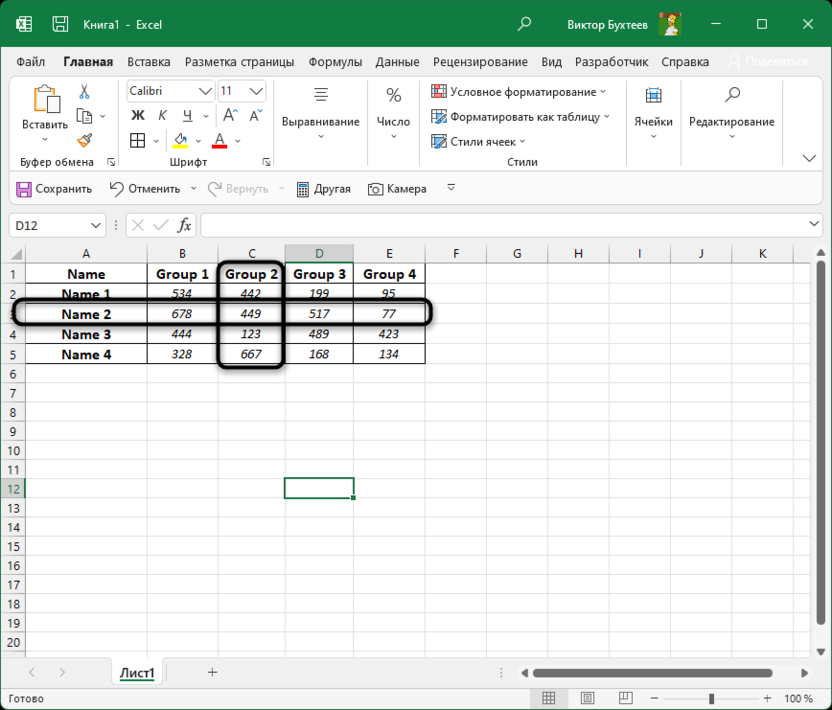## Standard usage of the intersection operator

To begin with, let’s figure out how to simply use the intersection operator and display the same desired value in an empty cell. You will need any table consisting of several columns and rows with populated data. Then it remains to perform the following actions:

1. Select an empty cell to display the result, add an = sign and select the entire row with the left mouse button.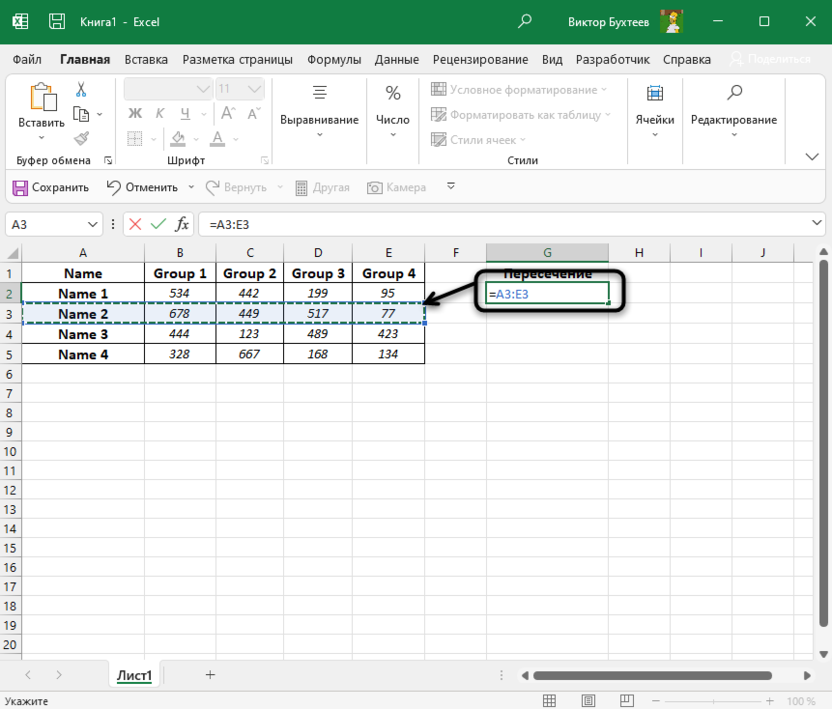2. After that, put a space. This sign is the intersection operator.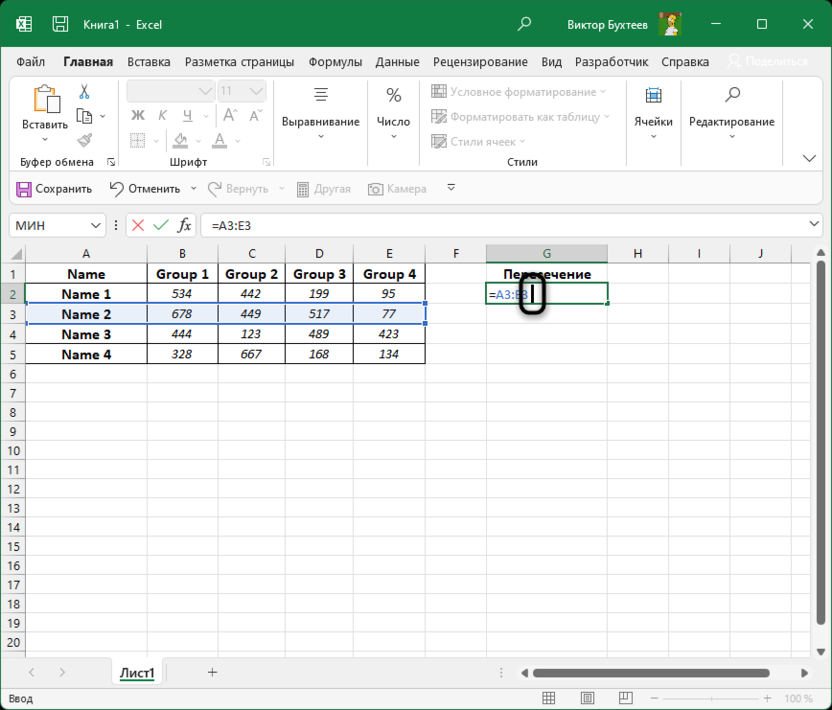3. After a space, specify the range of the column by also selecting it with the left mouse button.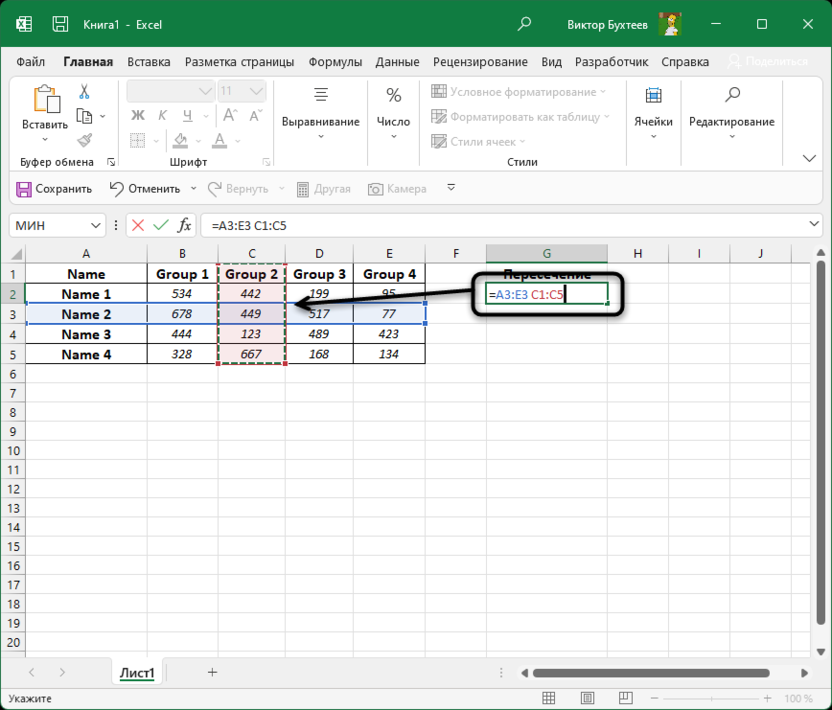4. Press the key Enterto see the result. As you can see, the operator found out which cell is in both the column and the row by displaying its value in the cell.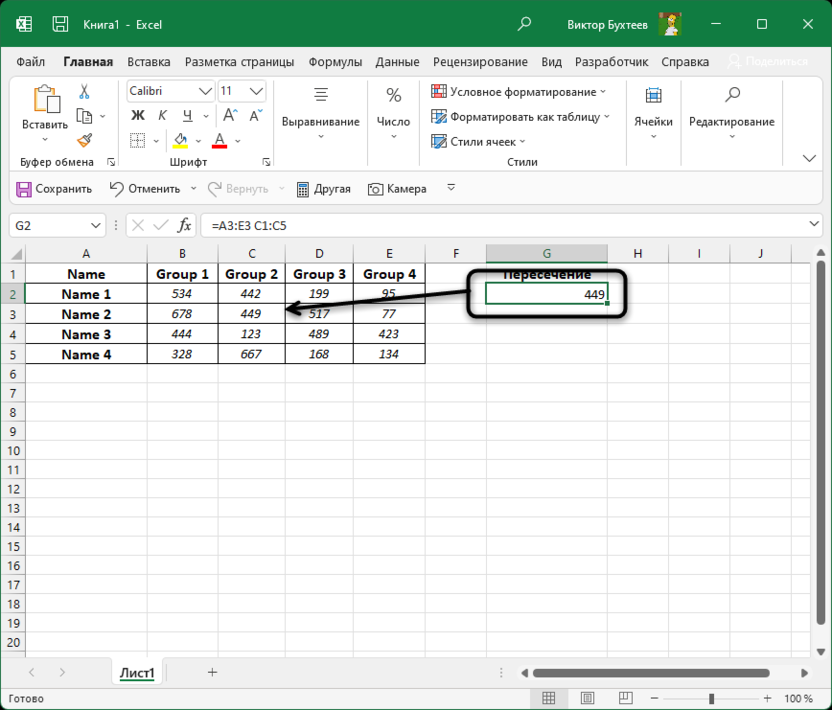The community is now in Telegram

Subscribe and stay up to date with the latest IT news

## Using the intersection operator in formulas

Although the formula described above with the use of the intersection operator seems interesting, it is practically not used in its pure form, since it is also clear from the outside what value is in the column and row. Most often, this operator is used together with various functions in calculations. You can make a row according to the example =SUM(A3:E3 C1:C5)+500so that the intersection operator finds a cell and adds another 500 to it. This is a fairly simple and standard operation.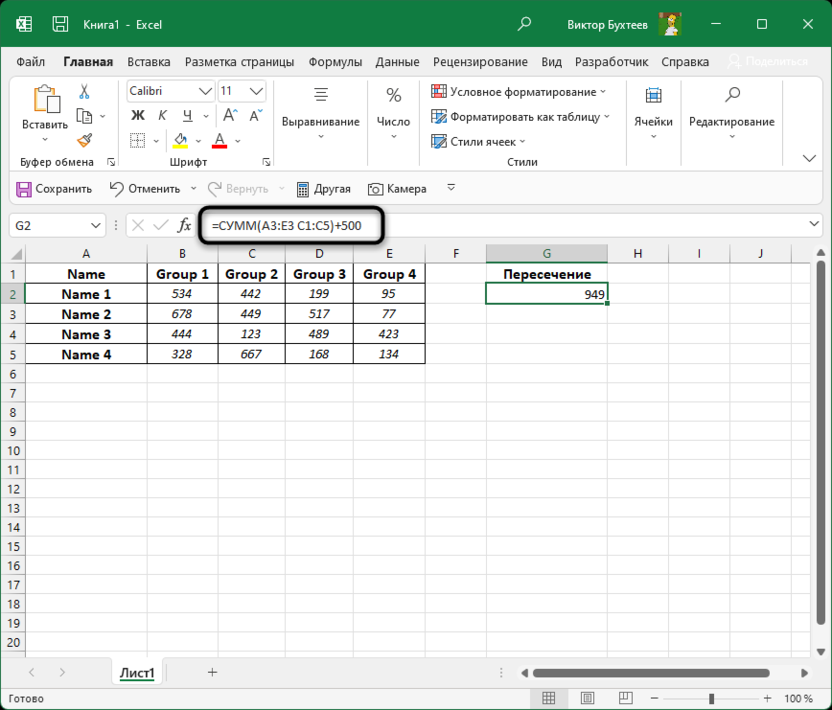About the same can be done with the function IF. A Boolean value uses the intersection operator and triggers a specific result of false or true that you configure yourself using the function arguments.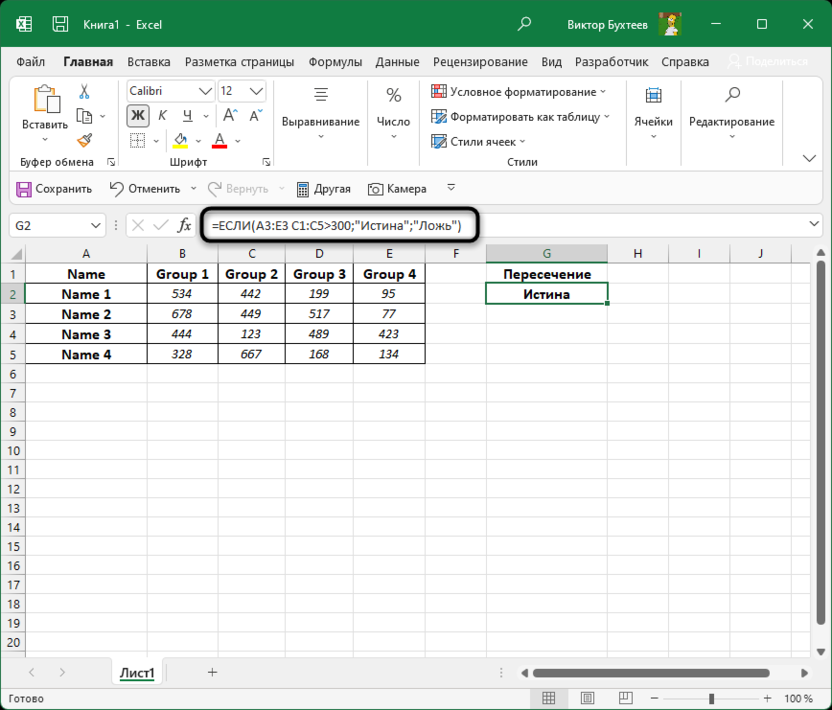In conclusion, we will consider a few more interesting points of working with the operator, which may be useful when performing calculations in a spreadsheet. For example, you can take a range consisting not only of one column and row, but also of several, sequentially selecting all the cells approximately as shown in the screenshot.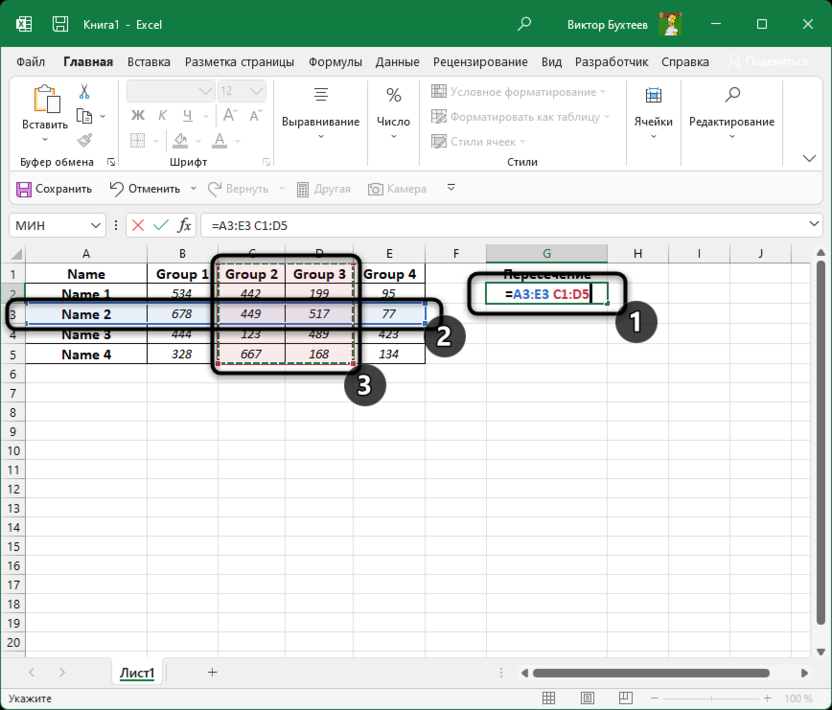By default, the intersection operator will not be able to output two or more values, so this range selection is only used with other functions. For example, you can sum all the values ​​that fit the intersection.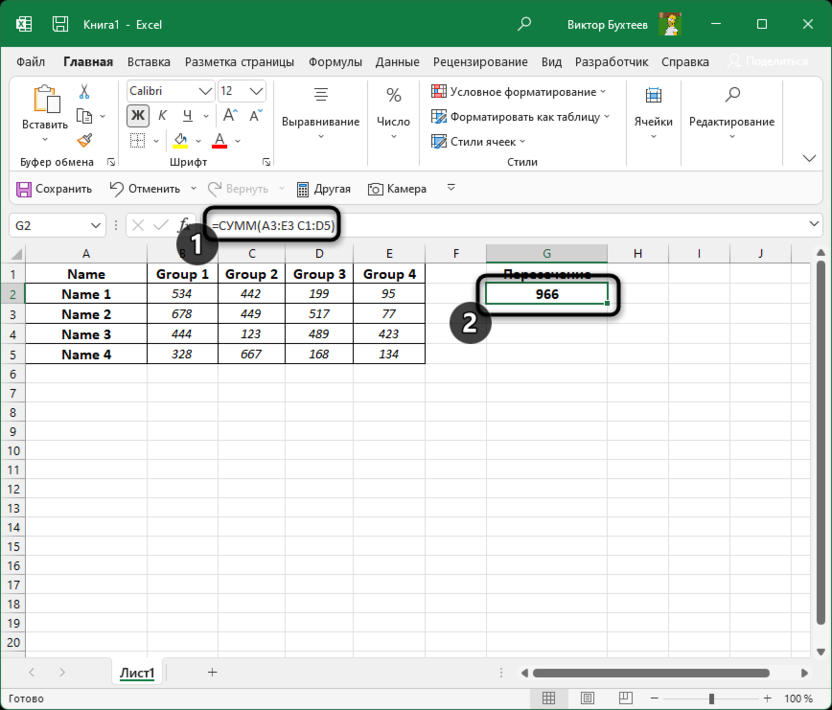Along with this, you can find the minimum value among them by applying a formula familiar to everyone MIN. Remember to only put a space between columns and rows, as this is the sign of the intersection operator.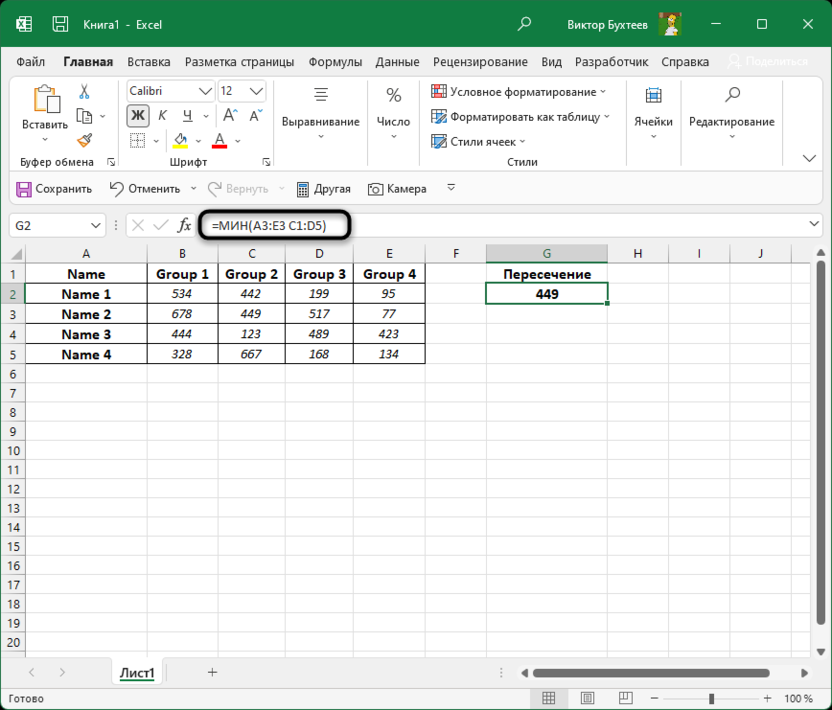If you are using a smart table or have given your own names for each column with rows, you can enter whole range names when selecting using the operator instead of the standard cell labels. The formula will then look something like the following image.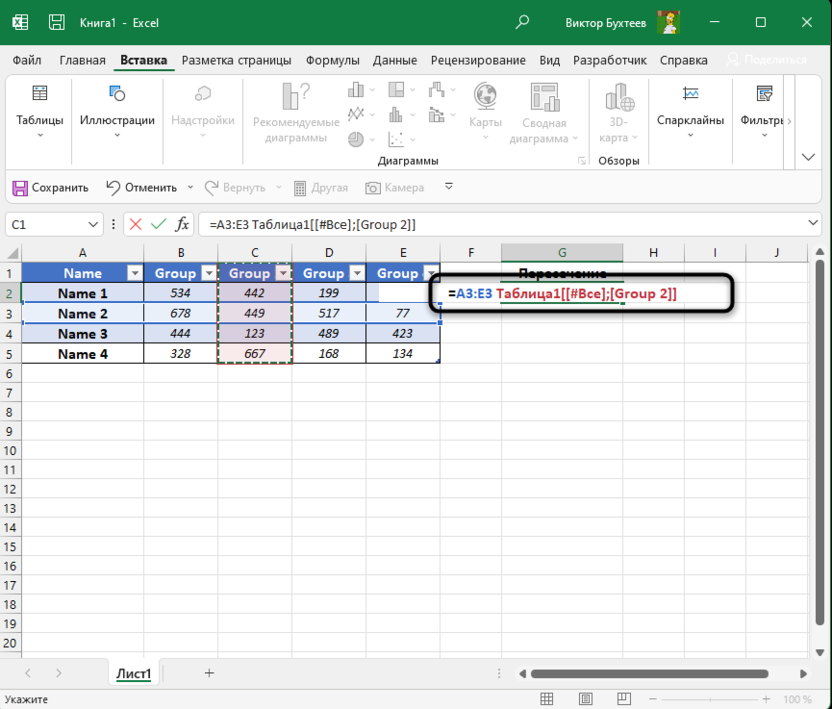## Finally

This is all and we have considered the basic principles of working with the intersection operator. I hope you can apply these skills in future calculations to simplify the process of working with spreadsheets.